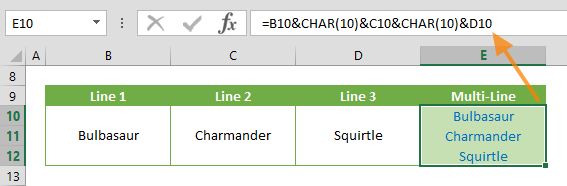The Excel CHAR formula is a Text function that returns a character, based on the character set used by your computer. In this guide, we’re going to show you how to use the Excel CHAR function and also go over some tips and error handling methods.

# Supported versions

• All Excel versions

CHAR(number)

# Arguments

 number A number between 1 and 255 specifying the character.

Examples

## Basics

Enter the character code for the argument value. Thus,

=CHAR(65)
formula returns the "A" character.## Insert a line break

You can create a line break by pressing the Alt + Enter key combination. However, this will not work for when you want to use the outcome of a formula. The CHAR function can be used for adding line breaks (or new lines) into a string that is generated by a formula.

For example, a formula like

=B10&CHAR(10)&C10&CHAR(10)&D10
combines the values from the three cells (B10, C10 and D10) and adds line breaks in between each value.Notes:

1. Remember to enable the Wrap Text option in the formula cell. Otherwise, Excel will display all values in a single row.
2. If you are using Mac, use =CHAR(13) instead. Windows and Mac OS use different character sets. See the Tips section below for more details.

# Tips

• The character codes will be different based on your operating system:
• Excel Online supports only 9, 10, 13, 32 and above
• Add 32 to the code of an uppercase alphabetic character to switch it to lowercase. For example, 65 = A, 97 = a.
• The CHAR function is great for inserting special characters that usually do not exist in keyboards. Some examples are as follows:
• Use the CODE function to return the code number of a character. The CODE function is essentially the opposite of the CHAR function.

# Issues

## #VALUE!

The function returns a #VALUE! error if the value is a string, or the reference cell is empty.